# Bias Mitigation in Credit Scoring by Disparate Impact Removal

Disparate impact removal is a pre-processing technique in bias mitigation. Using this technique, you modify the original credit score data to increase group fairness, while still preserving rank ordering within groups. Using a disparate impact removal technique reduces the bias introduced by the credit scoring model more than if you use the original data to train the credit scoring model. You perform the disparate impact removal technique using the `disparateImpactRemover` class from the Statistics and Machine Learning Toolbox™. This class returns a `remover` object along with a table containing the new predictor values. However, you need to use the `transform` method with the `remover` object on the test data before you can predict using the fitted credit scoring model.

The disparate impact removal technique works only with the distribution of data within a numeric predictor for each subgroup of a sensitive attribute. The `disparateImpactRemover` class has no knowledge of, or relation to, the response variable. In this example, you treat all the numeric predictors, time at address (`TmAtAddress`), customer income (`CustIncome`), time with Bank (`TmWBank`), average monthly balance (`AMBalance`), and utilization rate (`UtilRate`), with respect to the sensitive attribute, customer age (`AgeGroup`).

### Original Credit Scoring Model

This example uses a credit scoring workflow. Load the `CreditCardData.mat` and use the `'data'` data set.

```load CreditCardData.mat AgeGroup = discretize(data.CustAge,[min(data.CustAge) 30 45 60 max(data.CustAge)], ... 'categorical',{'Age < 30','30 <= Age < 45','45 <= Age < 60','Age >= 60'}); data = addvars(data,AgeGroup,'After','CustAge'); head(data)```
``` CustID CustAge AgeGroup TmAtAddress ResStatus EmpStatus CustIncome TmWBank OtherCC AMBalance UtilRate status ______ _______ ______________ ___________ __________ _________ __________ _______ _______ _________ ________ ______ 1 53 45 <= Age < 60 62 Tenant Unknown 50000 55 Yes 1055.9 0.22 0 2 61 Age >= 60 22 Home Owner Employed 52000 25 Yes 1161.6 0.24 0 3 47 45 <= Age < 60 30 Tenant Employed 37000 61 No 877.23 0.29 0 4 50 45 <= Age < 60 75 Home Owner Employed 53000 20 Yes 157.37 0.08 0 5 68 Age >= 60 56 Home Owner Employed 53000 14 Yes 561.84 0.11 0 6 65 Age >= 60 13 Home Owner Employed 48000 59 Yes 968.18 0.15 0 7 34 30 <= Age < 45 32 Home Owner Unknown 32000 26 Yes 717.82 0.02 1 8 50 45 <= Age < 60 57 Other Employed 51000 33 No 3041.2 0.13 0 ```
`rng('default')`

Split the data set into training and testing data.

```c = cvpartition(size(data,1),'HoldOut',0.3); data_Train = data(c.training(),:); data_Test = data(c.test(),:); head(data_Train)```
``` CustID CustAge AgeGroup TmAtAddress ResStatus EmpStatus CustIncome TmWBank OtherCC AMBalance UtilRate status ______ _______ ______________ ___________ __________ _________ __________ _______ _______ _________ ________ ______ 1 53 45 <= Age < 60 62 Tenant Unknown 50000 55 Yes 1055.9 0.22 0 2 61 Age >= 60 22 Home Owner Employed 52000 25 Yes 1161.6 0.24 0 3 47 45 <= Age < 60 30 Tenant Employed 37000 61 No 877.23 0.29 0 4 50 45 <= Age < 60 75 Home Owner Employed 53000 20 Yes 157.37 0.08 0 7 34 30 <= Age < 45 32 Home Owner Unknown 32000 26 Yes 717.82 0.02 1 8 50 45 <= Age < 60 57 Other Employed 51000 33 No 3041.2 0.13 0 9 50 45 <= Age < 60 10 Tenant Unknown 52000 25 Yes 115.56 0.02 1 10 49 45 <= Age < 60 30 Home Owner Unknown 53000 23 Yes 718.5 0.17 1 ```

Use `creditscorecard` to create a `creditscorecard` object and use `fitmodel` to fit a credit scoring model with the the training data (`data_Train`).

```PredictorVars = setdiff(data_Train.Properties.VariableNames, ... {'CustAge','AgeGroup','CustID','status'}); sc1 = creditscorecard(data_Train,'IDVar','CustID', ... 'PredictorVars',PredictorVars,'ResponseVar','status'); sc1 = autobinning(sc1); sc1 = fitmodel(sc1,'VariableSelection','fullmodel');```
```Generalized linear regression model: logit(status) ~ 1 + TmAtAddress + ResStatus + EmpStatus + CustIncome + TmWBank + OtherCC + AMBalance + UtilRate Distribution = Binomial Estimated Coefficients: Estimate SE tStat pValue ________ ________ ________ __________ (Intercept) 0.73924 0.077237 9.5711 1.058e-21 TmAtAddress 1.2577 0.99118 1.2689 0.20448 ResStatus 1.755 1.295 1.3552 0.17535 EmpStatus 0.88652 0.32232 2.7504 0.0059516 CustIncome 0.95991 0.19645 4.8862 1.0281e-06 TmWBank 1.132 0.3157 3.5856 0.00033637 OtherCC 0.85227 2.1198 0.40204 0.68765 AMBalance 1.0773 0.31969 3.3698 0.00075232 UtilRate -0.19784 0.59565 -0.33214 0.73978 840 observations, 831 error degrees of freedom Dispersion: 1 Chi^2-statistic vs. constant model: 66.5, p-value = 2.44e-11 ```

Use `displaypoints` to compute the points per predictor per bin for the `creditscorecard` model (`sc1`).

`pointsinfo1 = displaypoints(sc1)`
```pointsinfo1=38×3 table Predictors Bin Points _______________ _________________ _________ {'TmAtAddress'} {'[-Inf,9)' } -0.17538 {'TmAtAddress'} {'[9,16)' } 0.05434 {'TmAtAddress'} {'[16,23)' } 0.096897 {'TmAtAddress'} {'[23,Inf]' } 0.13984 {'TmAtAddress'} {'<missing>' } NaN {'ResStatus' } {'Tenant' } -0.017688 {'ResStatus' } {'Home Owner' } 0.11681 {'ResStatus' } {'Other' } 0.29011 {'ResStatus' } {'<missing>' } NaN {'EmpStatus' } {'Unknown' } -0.097582 {'EmpStatus' } {'Employed' } 0.33162 {'EmpStatus' } {'<missing>' } NaN {'CustIncome' } {'[-Inf,30000)' } -0.61962 {'CustIncome' } {'[30000,36000)'} -0.10695 {'CustIncome' } {'[36000,40000)'} 0.0010845 {'CustIncome' } {'[40000,42000)'} 0.065532 ⋮ ```

Use `probdefault` to determine the likelihood of default for the `data_Test` data set and the `creditscorecard` model (`sc1`).

```pd1 = probdefault(sc1,data_Test); threshold = 0.35; predictions1 = double(pd1>threshold);```

Use `fairnessMetrics` to compute fairness metrics at the model level as a baseline. Use `report` to generate the fairness metrics report.

```modelMetrics1 = fairnessMetrics(data_Test,'status','Predictions',predictions1,'SensitiveAttributeNames','AgeGroup'); mmReport1 = report(modelMetrics1,'GroupMetrics','GroupCount')```
```mmReport1=4×8 table ModelNames SensitiveAttributeNames Groups StatisticalParityDifference DisparateImpact EqualOpportunityDifference AverageAbsoluteOddsDifference GroupCount __________ _______________________ ______________ ___________________________ _______________ __________________________ _____________________________ __________ Model1 AgeGroup Age < 30 0.54312 2.6945 0.47391 0.5362 22 Model1 AgeGroup 30 <= Age < 45 0.19922 1.6216 0.35645 0.22138 152 Model1 AgeGroup 45 <= Age < 60 0 1 0 0 156 Model1 AgeGroup Age >= 60 -0.15385 0.52 -0.18323 0.16375 30 ```

Use `plot` to visualize the statistical parity difference (`'spd'`) and disparate impact (`'di'`) bias metrics.

```figure tiledlayout(2,1) nexttile plot(modelMetrics1,'spd') nexttile plot(modelMetrics1,'di')```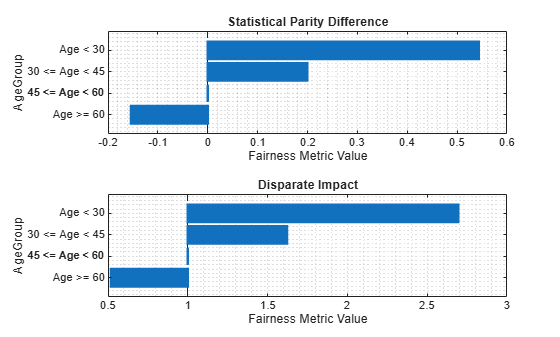### Bias Mitigation by Disparate Impact Removal

For each of the five continuous predictors, '`TmAtAddress'`, '`CustIncome'`, `'TmWBank'`, `'AMBalance'`, and `'UtilRate'` plot the original distributions of data within each age group.

Choose a numeric predictor to plot.

```predictor =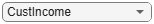"CustIncome"; [f1, xi1] = ksdensity(data_Train.(predictor)(data_Train.AgeGroup=='Age < 30')); [f2, xi2] = ksdensity(data_Train.(predictor)(data_Train.AgeGroup=='30 <= Age < 45')); [f3, xi3] = ksdensity(data_Train.(predictor)(data_Train.AgeGroup=='45 <= Age < 60')); [f4, xi4] = ksdensity(data_Train.(predictor)(data_Train.AgeGroup=='Age >= 60'));```

Create a `disparateImpactRemover` object and return the `newTrainTbl` table with the new predictor values.

`[remover, newTrainTbl] = disparateImpactRemover(data_Train, 'AgeGroup' , 'PredictorNames', {'TmAtAddress','CustIncome','TmWBank','AMBalance','UtilRate'})`
```remover = disparateImpactRemover with properties: RepairFraction: 1 PredictorNames: {'TmAtAddress' 'CustIncome' 'TmWBank' 'AMBalance' 'UtilRate'} SensitiveAttribute: 'AgeGroup' ```
```newTrainTbl=840×12 table CustID CustAge AgeGroup TmAtAddress ResStatus EmpStatus CustIncome TmWBank OtherCC AMBalance UtilRate status ______ _______ ______________ ___________ __________ _________ __________ _______ _______ _________ ________ ______ 1 53 45 <= Age < 60 58.599 Tenant Unknown 47000 51.733 Yes 1009.4 0.20099 0 2 61 Age >= 60 24 Home Owner Employed 41500 24.5 Yes 1203.9 0.25 0 3 47 45 <= Age < 60 30.5 Tenant Employed 33500 57.686 No 817.9 0.29 0 4 50 45 <= Age < 60 68.622 Home Owner Employed 49401 19.07 Yes 120.54 0.077267 0 7 34 30 <= Age < 45 30.5 Home Owner Unknown 35500 26.657 Yes 638.88 0.02 1 8 50 45 <= Age < 60 53.39 Other Employed 47000 27.971 No 2172.7 0.12 0 9 50 45 <= Age < 60 9 Tenant Unknown 49401 22.541 Yes 120.54 0.02 1 10 49 45 <= Age < 60 30.5 Home Owner Unknown 49401 22 Yes 664.51 0.14715 1 11 52 45 <= Age < 60 24 Tenant Unknown 30500 38.779 Yes 120.54 0.065 1 12 48 45 <= Age < 60 77.291 Other Unknown 40500 14.5 Yes 405.81 0.03 0 14 44 30 <= Age < 45 68.622 Other Unknown 44500 34.791 No 378.88 0.15657 0 17 39 30 <= Age < 45 9 Tenant Employed 37500 38.779 Yes 664.51 0.25 1 20 52 45 <= Age < 60 51.442 Other Unknown 38500 12.297 Yes 1157.5 0.19273 0 21 37 30 <= Age < 45 10.343 Tenant Unknown 36500 23.314 No 732.28 0.065 1 22 51 45 <= Age < 60 12.087 Home Owner Employed 31500 27.971 Yes 437.95 0.01 0 24 43 30 <= Age < 45 40 Tenant Employed 33500 11.18 Yes 263.13 0.077267 0 ⋮ ```
```[nf1, nxi1] = ksdensity(newTrainTbl.(predictor)(newTrainTbl.AgeGroup=='Age < 30')); [nf2, nxi2] = ksdensity(newTrainTbl.(predictor)(newTrainTbl.AgeGroup=='30 <= Age < 45')); [nf3, nxi3] = ksdensity(newTrainTbl.(predictor)(newTrainTbl.AgeGroup=='45 <= Age < 60')); [nf4, nxi4] = ksdensity(newTrainTbl.(predictor)(newTrainTbl.AgeGroup=='Age >= 60'));```

Plot the original and the repaired distributions.

```figure; tiledlayout(2,1) ax1 = nexttile; plot(xi1, f1, 'LineWidth', 1.5) hold on plot(xi2, f2, 'LineWidth', 1.5) plot(xi3, f3, 'LineWidth', 1.5) plot(xi4, f4, 'LineWidth', 1.5) legend(["Age < 30"; "30 <= Age < 45"; "45 <= Age < 60"; "Age >= 60"],'Location','northwest') ax1.Title.String = "Original Distribution of " + predictor; xlabel(predictor) ylabel('pdf') grid on ax2 = nexttile; plot(nxi1, nf1, 'LineWidth', 1.5) hold on plot(nxi2, nf2, 'LineWidth', 1.5) plot(nxi3, nf3, 'LineWidth', 1.5) plot(nxi4, nf4, 'LineWidth', 1.5) legend(["Age < 30"; "30 <= Age < 45"; "45 <= Age < 60"; "Age >= 60"],'Location','northwest') ax2.Title.String = "Repaired Distribution of " + predictor; xlabel(predictor) ylabel('pdf') grid on linkaxes([ax1, ax2], 'xy')```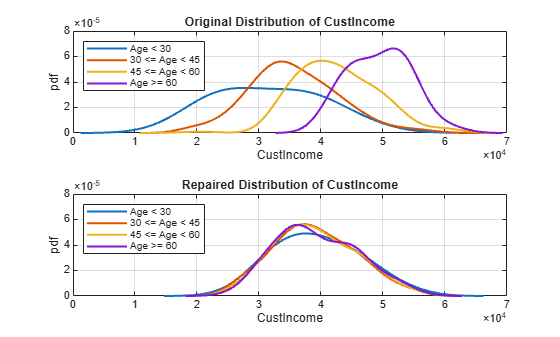This plot demonstrates that the initial distributions of `CustIncome` of each group within the `AgeGroup` predictor are different. Younger people seem to have a lower income on average and more variation than older people. This difference introduces bias, which the fitted model then reflects. The `disparateImpactRemover` function modifies the data so that the distributions of all the subgroups are more similar. You see this distribution in the second subplot Repaired Distribution of CustIncome. Using this new data, you can fit a logistic regression model and then measure the model-level metrics and compare these with the model-level metrics from the original `creditscorecard` model (`sc1`).

### New Credit Scoring Model

Use `creditscorecard` to create a `creditscorecard` object and use `fitmodel` to fit a credit scoring model with the the new data (`newTrainTbl`). Then, you can compute model-level bias metrics using `fairnessMetrics`.

```sc2 = creditscorecard(newTrainTbl,'IDVar','CustID', ... 'PredictorVars',PredictorVars,'ResponseVar','status'); sc2 = autobinning(sc2); sc2 = fitmodel(sc2,'VariableSelection','fullmodel');```
```Generalized linear regression model: logit(status) ~ 1 + TmAtAddress + ResStatus + EmpStatus + CustIncome + TmWBank + OtherCC + AMBalance + UtilRate Distribution = Binomial Estimated Coefficients: Estimate SE tStat pValue _________ _______ _______ __________ (Intercept) 0.74041 0.07641 9.6899 3.327e-22 TmAtAddress 1.1658 0.87564 1.3313 0.18308 ResStatus 1.8719 1.2848 1.4569 0.14513 EmpStatus 0.88699 0.31991 2.7727 0.00556 CustIncome 0.98269 0.28725 3.421 0.00062396 TmWBank 1.1392 0.30677 3.7135 0.00020442 OtherCC 0.55005 2.0969 0.26231 0.79308 AMBalance 1.0478 0.3607 2.9049 0.0036734 UtilRate -0.071972 0.58704 -0.1226 0.90242 840 observations, 831 error degrees of freedom Dispersion: 1 Chi^2-statistic vs. constant model: 50.4, p-value = 3.36e-08 ```

Use `displaypoints` to compute the points per predictor per bin for the `creditscorecard` model (`sc2`).

`pointsinfo2 = displaypoints(sc2)`
```pointsinfo2=35×3 table Predictors Bin Points _______________ _________________ _________ {'TmAtAddress'} {'[-Inf,9)' } -0.11003 {'TmAtAddress'} {'[9,15.1453)' } -0.091424 {'TmAtAddress'} {'[15.1453,Inf]'} 0.14546 {'TmAtAddress'} {'<missing>' } NaN {'ResStatus' } {'Tenant' } -0.024878 {'ResStatus' } {'Home Owner' } 0.11858 {'ResStatus' } {'Other' } 0.30343 {'ResStatus' } {'<missing>' } NaN {'EmpStatus' } {'Unknown' } -0.097539 {'EmpStatus' } {'Employed' } 0.3319 {'EmpStatus' } {'<missing>' } NaN {'CustIncome' } {'[-Inf,31500)' } -0.30942 {'CustIncome' } {'[31500,38500)'} -0.09789 {'CustIncome' } {'[38500,45000)'} 0.21233 {'CustIncome' } {'[45000,Inf]' } 0.494 {'CustIncome' } {'<missing>' } NaN ⋮ ```

Before computing probabilities of default with the test data, you need to transform the test data using the same transformation as for the training data. To make this transformation, use the `transform` method of the `remover` object and pass it in the `data_Test` data set. Then, use `probdefault` to compute the likelihood of default of the `data_Test` data set.

```newTestTbl = transform(remover,data_Test); pd2 = probdefault(sc2,newTestTbl); predictions2 = double(pd2>threshold);```

Use `fairnessMetrics` to compute fairness metrics at the model level and use `report` to generate a fairness metrics report.

```modelMetrics2 = fairnessMetrics(newTestTbl,'status','Predictions',predictions2,'SensitiveAttributeNames','AgeGroup'); mmReport2 = report(modelMetrics2,'GroupMetrics','GroupCount')```
```mmReport2=4×8 table ModelNames SensitiveAttributeNames Groups StatisticalParityDifference DisparateImpact EqualOpportunityDifference AverageAbsoluteOddsDifference GroupCount __________ _______________________ ______________ ___________________________ _______________ __________________________ _____________________________ __________ Model1 AgeGroup Age < 30 0.082751 1.2226 0.18696 0.10408 22 Model1 AgeGroup 30 <= Age < 45 -0.0033738 0.99093 0.07902 0.076333 152 Model1 AgeGroup 45 <= Age < 60 0 1 0 0 156 Model1 AgeGroup Age >= 60 0.028205 1.0759 0.015528 0.026143 30 ```

Use `plot` to visualize the statistical parity difference (`'spd'`) and disparate impact (`'di'`) bias metrics.

```figure tiledlayout(2,1) nexttile plot(modelMetrics2,'spd') nexttile plot(modelMetrics2,'di')```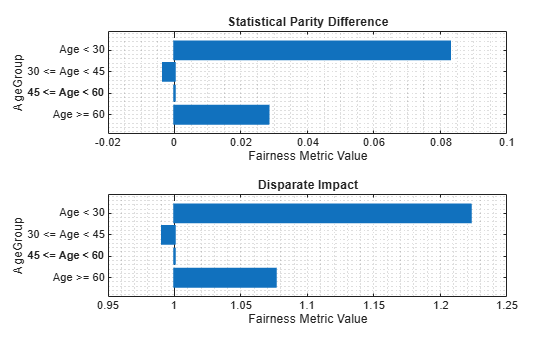### Plot Disparate Impact and Accuracy for Different Repair Fraction Values

In this example, the bias mitigation process uses `disparateImpactRemover` to set `RepairFraction` `= 1` in order to mitigate bias. However, it is useful to see how the disparate impact and accuracy varies with a change in the `RepairFraction` value. For example, use the `AgeGroup` predictor and plot the disparate impact and accuracy of the different subgroups for different values of `RepairFraction`.

```subgroup =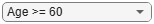4; r = 0:0.1:1; Accuracy = zeros(11,1); di = zeros(11,1); for i = 0:1:10 [rmvr, trainTbl] = disparateImpactRemover(data_Train, 'AgeGroup' , ... 'PredictorNames', {'TmAtAddress','CustIncome','TmWBank','AMBalance','UtilRate'},'RepairFraction',i/10); testTbl = transform(rmvr, data_Test); sc = creditscorecard(trainTbl,'IDVar','CustID', ... 'PredictorVars',PredictorVars,'ResponseVar','status'); sc = autobinning(sc); sc = fitmodel(sc,'VariableSelection','fullmodel','Display','off'); pd = probdefault(sc,testTbl); predictions = double(pd>threshold); modelMetrics = fairnessMetrics(newTestTbl, 'status', 'Predictions', predictions, 'SensitiveAttributeNames','AgeGroup'); mmReport = report(modelMetrics,'BiasMetrics','di','GroupMetrics','accuracy'); di(i+1) = mmReport.DisparateImpact(subgroup); Accuracy(i+1) = mmReport.Accuracy(subgroup); end figure yyaxis left plot(r, di,'LineWidth', 1.5) title('Bias Mitigation in AgeGroup ') xlabel('Repair Fraction') ylabel('Disparate Impact') yyaxis right plot(r, Accuracy,'LineWidth', 1.5) ylabel('Accuracy') grid on```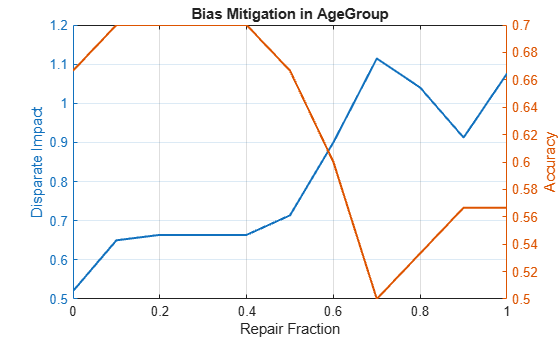If you select the subgroup `'Age < 30'` from this plot, you can see that the accuracy increases as the `RepairFraction` value increases. Although this seems counterintuitive, looking further at the `GroupCount` of that age group in the `mmReport2` table, this group has only 22 observations. This small number of observations explains the anomaly in this plot.

One way to mitigate this issue of not having enough data for a subgroup is to combine all unprivileged groups and compare them as one group against the privileged group. The following code shows you how by setting the majority group (`45 <= Age < 60`) as the privileged group and then by combining every other group into one and setting that group as the unprivliged group.

```privilegedGroup = '45 <= Age < 60'; twoAgeGroups_TrainTbl = data_Train; twoAgeGroups_TrainTbl.AgeGroup = addcats(twoAgeGroups_TrainTbl.AgeGroup,'Other','After','Age >= 60'); twoAgeGroups_TrainTbl.AgeGroup(twoAgeGroups_TrainTbl.AgeGroup ~= privilegedGroup) = 'Other'; twoAgeGroups_TestTbl = data_Test; twoAgeGroups_TestTbl.AgeGroup = addcats(twoAgeGroups_TestTbl.AgeGroup,'Other','After','Age >= 60'); twoAgeGroups_TestTbl.AgeGroup(twoAgeGroups_TestTbl.AgeGroup ~= privilegedGroup) = 'Other'; r = 0:0.1:1; Accuracy = zeros(11,1); di = zeros(11,1); for i = 0:1:10 [rmvr, trainTbl] = disparateImpactRemover(twoAgeGroups_TrainTbl, 'AgeGroup' , ... 'PredictorNames', {'TmAtAddress','CustIncome','TmWBank','AMBalance','UtilRate'},'RepairFraction',i/10); testTbl = transform(rmvr, twoAgeGroups_TestTbl); sc = creditscorecard(trainTbl,'IDVar','CustID', ... 'PredictorVars',PredictorVars,'ResponseVar','status'); sc = autobinning(sc); sc = fitmodel(sc,'VariableSelection','fullmodel','Display','off'); pd = probdefault(sc,testTbl); predictions = double(pd>threshold); modelMetrics = fairnessMetrics(twoAgeGroups_TestTbl, 'status', 'Predictions', predictions, ... 'SensitiveAttributeNames','AgeGroup','ReferenceGroup','45 <= Age < 60'); mmReport = report(modelMetrics,'BiasMetrics','di','GroupMetrics','accuracy'); di(i+1) = mmReport.DisparateImpact(2); Accuracy(i+1) = mmReport.Accuracy(2); end figure yyaxis left plot(r, di,'LineWidth', 1.5) title('Bias Mitigation in AgeGroup ') xlabel('Repair Fraction') ylabel('Disparate Impact') yyaxis right plot(r, Accuracy,'LineWidth', 1.5) ylabel('Accuracy') grid on```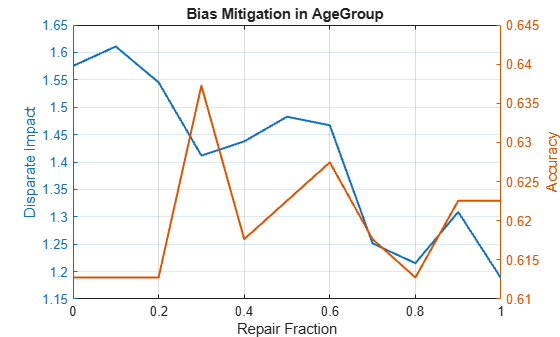You can use this privileged group and unprivliged group method if the goal is not to measure the bias of each individual group against the privileged group, but rather to measure the overall fairness of all customers who are not part of the privileged group.

### References

 Nielsen, Aileen. "Chapter 4. Fairness PreProcessing." Practical Fairness. O'Reilly Media, Inc., Dec. 2020.

 Mehrabi, Ninareh, et al. “A Survey on Bias and Fairness in Machine Learning.” ArXiv:1908.09635 [Cs], Sept. 2019. arXiv.org, https://arxiv.org/abs/1908.09635.

 Wachter, Sandra, et al. Bias Preservation in Machine Learning: The Legality of Fairness Metrics Under EU Non-Discrimination Law. SSRN Scholarly Paper, ID 3792772, Social Science Research Network, 15 Jan. 2021. papers.ssrn.com, https://papers.ssrn.com/sol3/papers.cfm?abstract_id=3792772.